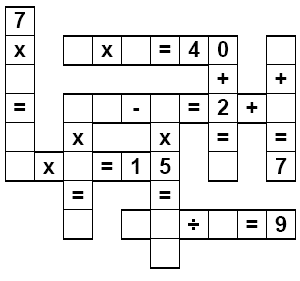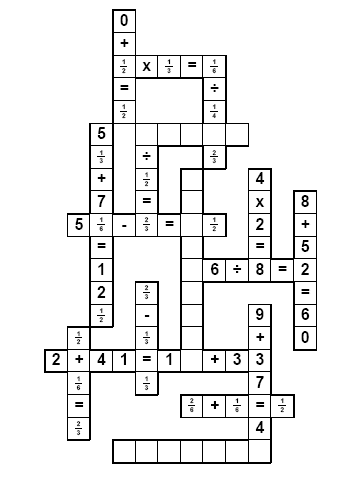Worksheets and No Prep Teaching Resources Runaway Math UnitFifth and Sixth GradeRunaway Math

Runaway Math Puzzles
Fifth and sixth grade runaway math puzzles
Runaway math puzzles with fractions

Create a custom Runaway Math Puzzle
Create a custom runaway math puzzle
Create a custom runaway math puzzle with missing equations
Create a custom runaway math puzzle where everything is missing

Removing Two Numbers from Equations - No FractionsAll of the equations are each missing at least one number (easy; most are automatic)
Large puzzle with three missing equations
Ten missing pieces
Fifteen missing pieces
Three of the equations are each missing at least two numbers
All of the equations are each missing at least two numbers (difficult)
All of the equations are each missing at least two numbers (larger puzzle; even more difficult!)
Everything missing except for operators (very challenging; as some may say "good luck!")

Multiplication and Division Puzzles
All of the equations are each missing at least one number (easy; most are automatic)
Large puzzle with three missing equations
Ten missing pieces
Fifteen missing pieces
Three of the equations are each missing at least two numbers
All of the equations are each missing at least two numbers (difficult)
All of the equations are each missing at least two numbers (larger puzzle; even more difficult!)
Everything missing except for operators (very challenging; as some may say "good luck!")

Addition, Subtraction, Multiplication, and Division Puzzles
All of the equations are each missing at least one number (easy; most are automatic)
Large puzzle with three missing equations
Ten missing pieces
Fifteen missing pieces
Three of the equations are each missing at least two numbers
All of the equations are each missing at least two numbers (difficult)
All of the equations are each missing at least two numbers (larger puzzle; even more difficult!)
Everything missing except for operators (very challenging; as some may say "good luck!")

Runaway Math Puzzles: Extra pieces given on second page - No Fractions
(students will need to ignore some of the extra pieces)

Addition and Subtraction Puzzles (with extra pieces)
Five numbers missing (one extra piece)
Seven numbers missing (one extra piece)
Nine numbers missing (two extra pieces)
Two equations missing (one extra piece)
Three equations missing (two extra pieces)
Seven numbers or operators missing (one extra piece)
Nine numbers or operators missing (one extra piece)
20 % of puzzle missing (one extra piece)
20 % of puzzle missing and all operators (one extra piece)
40 % of puzzle missing (two extra pieces)

Multiplication and Division Puzzles (with extra pieces)
Five numbers missing (one extra piece)
Seven numbers missing (one extra piece)
Nine numbers missing (two extra pieces)
Two equations missing (one extra piece)
Three equations missing (two extra pieces)
Seven numbers or operators missing (one extra piece)
Nine numbers or operators missing (one extra piece)
20 % of puzzle missing (one extra piece)
20 % of puzzle missing and all operators (one extra piece)
40 % of puzzle missing (two extra pieces)

Addition, Subtraction, Multiplication, and Division Puzzles (with extra pieces)
Five numbers missing (one extra piece)
Seven numbers missing (one extra piece)
Nine numbers missing (two extra pieces)
Two equations missing (one extra piece)
Three equations missing (two extra pieces)
Seven numbers or operators missing (one extra piece)
Nine numbers or operators missing (one extra piece)
20 % of puzzle missing (one extra piece)
20 % of puzzle missing and all operators (one extra piece)
40 % of puzzle missing (two extra pieces)

Removing Two Numbers from Equations - With FractionsFractions: All of the equations are each missing at least one number (easy; most are automatic)
Fractions: Large puzzle with three missing equations
Fractions: Ten missing pieces
Fractions: Fifteen missing pieces
Fractions: Three of the equations are each missing at least two numbers
Fractions: All of the equations are each missing at least two numbers (difficult)
Fractions: All of the equations are each missing at least two numbers (larger puzzle; even more difficult!)
Fractions: Everything missing except for operators (very challenging; as some may say "good luck!")

Multiplication and Division Puzzles
Fractions: All of the equations are each missing at least one number (easy; most are automatic)
Fractions: Large puzzle with three missing equations
Fractions: Ten missing pieces
Fractions: Fifteen missing pieces
Fractions: Three of the equations are each missing at least two numbers
Fractions: All of the equations are each missing at least two numbers (difficult)
Fractions: All of the equations are each missing at least two numbers (larger puzzle; even more difficult!)
Fractions: Everything missing except for operators (very challenging; as some may say "good luck!")

Addition, Subtraction, Multiplication, and Division Puzzles
Fractions: All of the equations are each missing at least one number (easy; most are automatic)
Fractions: Large puzzle with three missing equations
Fractions: Ten missing pieces
Fractions: Fifteen missing pieces
Fractions: Three of the equations are each missing at least two numbers
Fractions: All of the equations are each missing at least two numbers (difficult)
Fractions: All of the equations are each missing at least two numbers (larger puzzle; even more difficult!)
Fractions: Everything missing except for operators (very challenging; as some may say "good luck!")

Runaway Math Puzzles: Extra pieces given on second page - With Fractions
(students will need to ignore some of the extra pieces)

Addition and Subtraction Puzzles (with extra pieces)
Fractions: Five numbers missing (one extra piece)
Fractions: Seven numbers missing (one extra piece)
Fractions: Nine numbers missing (two extra pieces)
Fractions: Two equations missing (one extra piece)
Fractions: Three equations missing (two extra pieces)
Fractions: Seven numbers or operators missing (one extra piece)
Fractions: Nine numbers or operators missing (one extra piece)
Fractions: 20 % of puzzle missing (one extra piece)
Fractions: 20 % of puzzle missing and all operators (one extra piece)
Fractions: 40 % of puzzle missing (two extra pieces)

Multiplication and Division Puzzles (with extra pieces)
Fractions: Five numbers missing (one extra piece)
Fractions: Seven numbers missing (one extra piece)
Fractions: Nine numbers missing (two extra pieces)
Fractions: Two equations missing (one extra piece)
Fractions: Three equations missing (two extra pieces)
Fractions: Seven numbers or operators missing (one extra piece)
Fractions: Nine numbers or operators missing (one extra piece)
Fractions: 20 % of puzzle missing (one extra piece)
Fractions: 20 % of puzzle missing and all operators (one extra piece)
Fractions: 40 % of puzzle missing (two extra pieces)

Addition, Subtraction, Multiplication, and Division Puzzles (with extra pieces)
Fractions: Five numbers missing (one extra piece)
Fractions: Seven numbers missing (one extra piece)
Fractions: Nine numbers missing (two extra pieces)
Fractions: Two equations missing (one extra piece)
Fractions: Three equations missing (two extra pieces)
Fractions: Seven numbers or operators missing (one extra piece)
Fractions: Nine numbers or operators missing (one extra piece)
Fractions: 20 % of puzzle missing (one extra piece)
Fractions: 20 % of puzzle missing and all operators (one extra piece)
Fractions: 40 % of puzzle missing (two extra pieces)

Have a suggestion or would like to leave feedback?